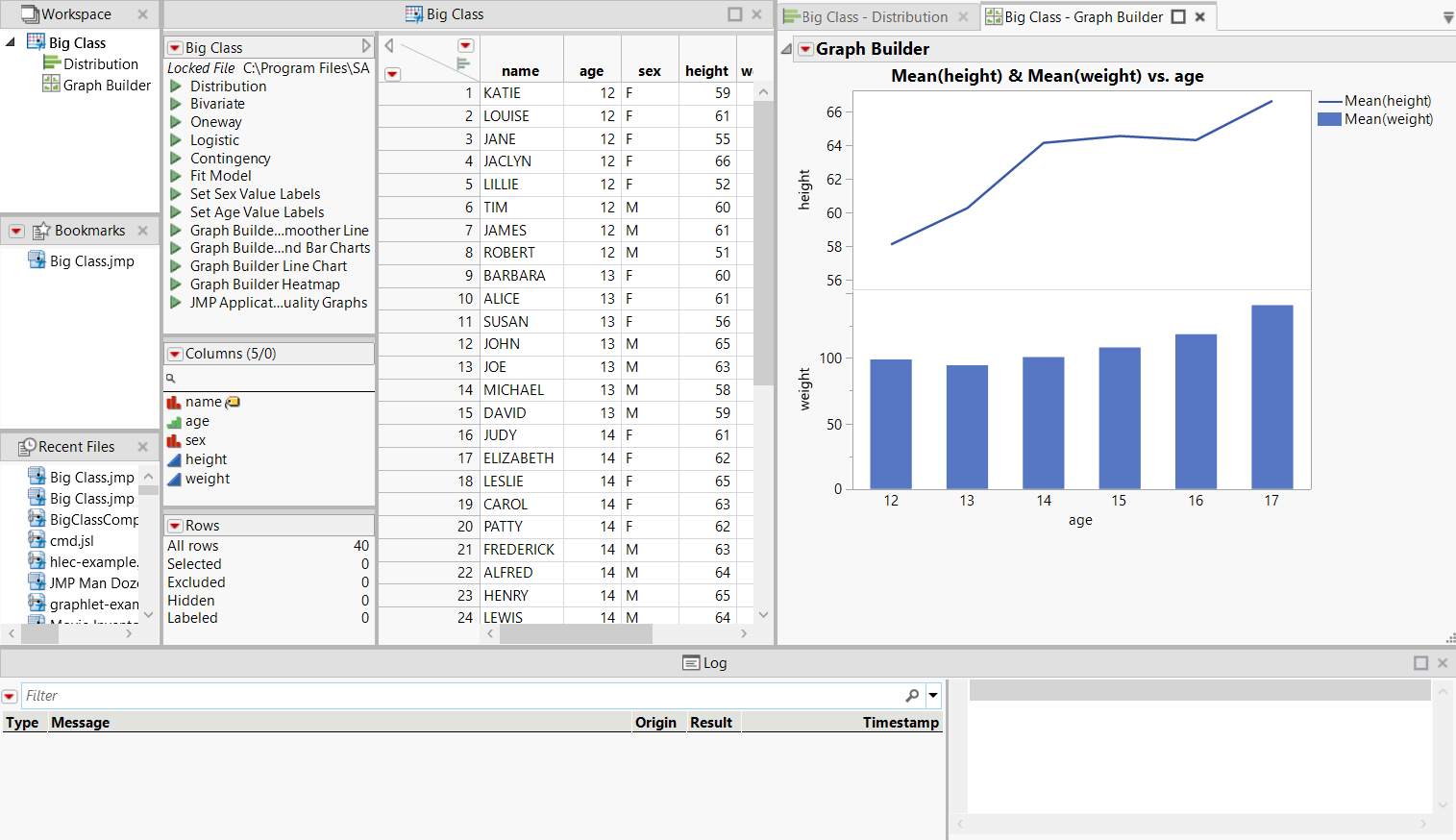Scripting Guide > Creating Projects > Create a Project with a Bookmarks Pane and Log
Publication date: 11/10/2021

# Create a Project with a Bookmarks Pane and Log

A more complicated project might include a Bookmarks pane (to show file, folders, and groups that use frequently), and a project log. The data table and output are in separate tabs.

Begin by creating a new project and creating a bookmark.

`project = New Project();`
`project << Add Bookmarks({`
`	File( "\$SAMPLE_DATA/Big Class.jmp" )`
`});`

Run Script opens the data table and creates output inside the project.

`project << Run Script(`
`	Open(`
`		"\$SAMPLE_DATA/Big Class.jmp",`
`		Set Window ID( "data" ) // unique window ID`
`	);`
`	Distribution(`
`		Set Window ID( "distrib" ),`
`		Continuous Distribution( Column( :weight ) ),`
`		Nominal Distribution( Column( :age ) )`
`	);`
`	Graph Builder(`
`		Set Window ID( "graphbuilder" ),`
`		Size( 443, 409 ),`
`		Show Control Panel( 0 ),`
`		Fit to Window( "Maintain Aspect Ratio" ),`
`		Variables( X( :age ), Y( :height ), Y( :weight ) ),`
`		Elements( Position( 1, 1 ), Line( X, Y, Legend( 1 ) ) ),`
`		Elements( Position( 1, 2 ), Bar( X, Y, Legend( 2 ) ) )`
`	);`
`);`

Set Layout specifies the layout of the tabs that show the data table and output.

`project << Set Layout(`
`	V Splitter Box(`
`		Size( 1215, 700 ),`
`		<<Set Sizes( {0.77, 0.23} ),`
`		<<Dockable(),`
`		V Splitter Box(`
`			Size( 1215, 540 ),`
`			<<Set Sizes( {1} ),`
`			<<Dockable(),`
`			H Splitter Box(`
`				Size( 1215, 540 ),`
`				<<Set Sizes( {0.11, 0.42, 0.47} ),`
`				<<Dockable(),`
`				V Splitter Box(`
`					Size( 128, 540 ),`
`					<<Set Sizes( {0.33, 0.33, 0.33} ),`
`					<<Dockable(),`
`					Tab Page Box(`
`						Title( "Window List" ), // show the Window List`
`						Window ID( "Window List" )`
`					),`
`					Tab Page Box(`
`						Title( "Bookmarks" ), // show the Bookmarks pane`
`						Window ID( "Bookmarks" )`
`					),`
`					Tab Page Box(`
`						Title( "Recent Files" ), // show the Recent Files pane`
`						Window ID( "Recent Files" )`
`					)`
`				),`
`				Tab Page Box( // show the data table`
`					Title( "Big Class" ),`
`					Window ID( "data" )`
`				),`
`				Tab Box(`
`					<<Dockable(),`
`					Tab Page Box( // show the Distribution graph and reports`
`						Title( "Big Class - Distribution" ),`
`						Window ID( "distrib" )`
`					),`
`					Tab Page Box( // show the Graph Builder graph`
`						Title( "Big Class - Graph Builder" ),`
`						Window ID( "graphbuilder" )`
`					)`
`				)`
`			)`
`		),`
`		Tab Page Box( Title( "Log" ), Window ID( "Log" ) )`
`	)`
`);`

Save saves the project.

// if the project has already been saved, the Save message

// with no argument saves the file to the existing location

`project << Save( "\$DOCUMENTS/Big Class.jmpprj" );`

Note: If you're saving a project with unsaved documents in JSL, the project doesn't close and you get a log message that the project has unsaved documents. All files in a project must be saved or closed before the project can be saved. However, if you interactively save a project with unsaved documents, you are prompted to save the documents.

Figure 15.2 Example of a Complex ProjectOpen the project from another script:

`project = Open( "Big Class.jmpprj" );`

In this example, the script that you are running to open the project is in the same folder as the project.